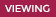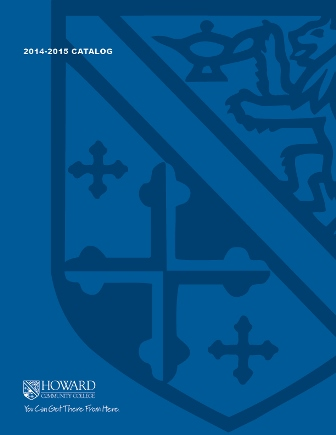2019-2020 College Catalog# MATH 181 Calculus I

Students will develop skills in the initial content of both differential and integral calculus including finding limits of functions; exposure to the epsilon-delta process and continuity; finding derivatives of polynomial, rational, radical, trigonometric, inverse trigonometric, exponential, and logarithmic functions, and inverse functions; the chain rule; and integration by substitution. Applications dealing with optimization, related rates, Newton's method, L'Hopital's rule, motion problems and the properties of the graphs of functions are covered. Theorems include the mean-value theorem for derivatives and integrals, the squeeze theorem, and the fundamental theorems of calculus. Implicit differentiation, differentials and summations of area will be used when appropriate. The use of a graphing calculator will be an integral part of the course. Credit will only be granted for one of the following: MATH 140, MATH 145, or MATH 181.

4

### Prerequisite

MATH 153 or MATH 155 with a grade of C or higher, or appropriate score on the mathematics placement test

4 hours weekly

### Course Objectives

1. Calculate limits of elementary functions numerically, graphically, and analytically.
2. Calculate derivatives of functions given either implicitly or explicitly.
3. Apply the method of substitution to calculate integrals of functions where applicable.
4. Apply the theory of derivatives to the graphing of curves and to solve related rate and
optimization problems, and estimation of zeroes of a function with Newton’s method.
5. Use differential calculus to model and solve various application problems, interpret results,
and evaluate the reasonableness of the results in context.
6. Apply the Mean Value Theorem for derivatives and integrals to given functions.
7. Use a computer algebra system, as a means of discovery, to reinforce concepts, and as an
efficient problem-solving tool.
8. Communicate calculus concepts in a neat and organized manner using appropriate
symbols, notation, and vocabulary.
9. Identify and implement appropriate technologies to efficiently complete tasks that involve the
solving of cross-discipline, mathematically appropriate problems and creating new works to
communicate the processes used and solution.

### Course Objectives

Calculate limits of elementary functions numerically, graphically, and analytically.

This objective is a course Goal Only

Calculate derivatives of functions given either implicitly or explicitly.

This objective is a course Goal Only

Apply the method of substitution to calculate integrals of functions where applicable.

This objective is a course Goal Only

Apply the theory of derivatives to the graphing of curves and to solve related rate and
optimization problems, and estimation of zeroes of a function with Newton’s method.

#### Learning Activity Artifact

• Other (please fill out box below)
• Exam 2 (graphing and Newton's Method), Final exams (Related Rates and Optimization)

#### Procedure for Assessing Student Learning

• Scientific Reasoning Rubric

Use differential calculus to model and solve various application problems, interpret results,
and evaluate the reasonableness of the results in context.

#### Learning Activity Artifact

• Other (please fill out box below)
• Final exam (related rates and optimization)

#### Procedure for Assessing Student Learning

• Scientific Reasoning Rubric

Apply the Mean Value Theorem for derivatives and integrals to given functions.

This objective is a course Goal Only

Use a computer algebra system, as a means of discovery, to reinforce concepts, and as an
efficient problem-solving tool.

This objective is a course Goal Only

Communicate calculus concepts in a neat and organized manner using appropriate
symbols, notation, and vocabulary.

#### Learning Activity Artifact

• Other (please fill out box below)
• Final exam, project

#### Procedure for Assessing Student Learning

• Scientific Reasoning Rubric

Identify and implement appropriate technologies to efficiently complete tasks that involve the
solving of cross-discipline, mathematically appropriate problems and creating new works to
communicate the processes used and solution.

#### Learning Activity Artifact

• Other (please fill out box below)
• Projects

#### Procedure for Assessing Student Learning

• Technological Literacy Rubric